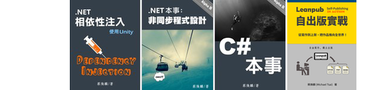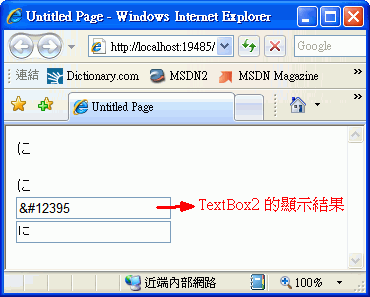2-25 Update經網友 Laneser 提醒，其實這篇提到的問題用 HtmlDecode 就簡單解決掉啦! 裡面提到的函式大概就只剩下 FindUnicodeEntities 可能還有用處吧! 其他一長串的東西就不用浪費時間看啦!

GirdView 還好辦，TextBox 控制項就麻煩了，因為它預設會自動處理 HTML 編碼形式的 Unicode 字串，卻沒有提供 HtmlEncode 屬性。以下是一個簡單的 ASP.NET 範例程式，可以測試這個問題：

`   1:  protected void Page_Load(object sender, EventArgs e)`
`   2:  {`
`   3:      string s = "&#12395;";            // 'に'`
`   4:      Response.Write(s + "<BR/>");    // OK!'`
`   5:      Label1.Text = s;                // OK!'`
`   6:          `
`   7:      // TextBox 內建 HTML encoding 功能，反而無法秀出 unicode 代碼所表示的字元.`
`   8:      TextBox1.Text = s;    `
```   9:
```
`  10:      // 經過轉換就 ok 了.`
`  11:      TextBox2.Text = Convert.ToChar(Convert.ToInt32(s.Substring(2, 5))).ToString();`
`  12:      }``   1:      /// <summary>`
`   2:      /// 將 Unicode 的 HTML 碼轉換成 Unicode 字元。`
`   3:      /// <para>C# 範例：<</para>`
`   4:      /// <p>char ch = UnicodeEntityToChar("&#12395;");`
`   5:      /// </p>`
`   6:      /// </summary>`
`   7:      /// <param name="entity">Unicode 的 HTML 碼。</param>`
`   8:      /// <returns>字元。</returns>`
`   9:      public static char UnicodeEntityToChar(string entity)`
`  10:      {`
`  11:          int code = Convert.ToInt32(entity.Substring(2, entity.Length - 3));`
`  12:          return Convert.ToChar(code);`
`  13:      }`
```  14:
```
`  15:      /// <summary>`
`  16:      /// 找出指定字串中的所有 Unicode HTML 碼。`
`  17:      /// </summary>`
`  18:      /// <param name="s">輸入字串。</param>`
`  19:      /// <returns>所有找到的 Unicode HTML 碼的集合。</returns>`
`  20:      public static MatchCollection FindUnicodeEntities(string s)`
`  21:      {`
`  22:          string pattern = @"&\#[0-9]{1,5};";`
`  23:          Regex regex = new Regex(pattern);`
`  24:          MatchCollection matches = regex.Matches(s);`
`  25:          return matches;`
`  26:      }`
```  27:
```
```  28:
```
`  29:      /// <summary>`
`  30:      /// 將傳入字串中的 Unicode 字碼（&#00000 格式）轉換成實際的 Unicode 字元。`
`  31:      /// </summary>`
`  32:      /// <param name="s">傳入字串。</param>`
`  33:      /// <returns>已轉換完成的字串。</returns>`
`  34:      public static string ReplaceUnicodeEntityToChar(string s)`
`  35:      {`
`  36:          StringBuilder result = new StringBuilder(s);`
`  37:          MatchCollection matches = FindUnicodeEntities(s);`
```  38:
```
`  39:          // Note: 不可從第一個符合的項目開始替換字串，須倒著處理.`
`  40:          for (int i = matches.Count-1; i >= 0; i--) `
`  41:          {`
`  42:              Match m = matches[i];`
`  43:              result.Remove(m.Index, m.Length);`
`  44:              result.Insert(m.Index, UnicodeEntityToChar(m.Value));`
`  45:          }`
`  46:          return result.ToString();`
`  47:      }`

`   1:  string test = "這是日文：&#12395;，再一個：&#12395;，再兩個：&#12395;&#12395;";`
`   2:  string s = ReplaceUnicodeEntityToChar(test);`
`   3:  Response.Write(s + "<BR/>") ;`

Happy coding :)

#### 4 則留言:

1.why not use HttpUtility.HtmlDecode ??

2.Hi Laneser,
對耶！我怎麼都沒想到 HtmlEncode 對應的就是 HtmlDecode 啊！蠢極了 @_@||

這篇文章應該刪掉的，但我想還是留著，為我這次的愚蠢作個紀念和警惕吧!

Thanks!!

3.格主太精實了, 讓我忍不住拿壓箱寶跟格主獻醜, 以下是我做的類似 code:

private static Regex reg = new Regex(@"&\#([0-9]{1,5});", RegexOptions.Compiled);

public static string HtmlDecode(string content)
{
return reg.Replace(content, m => Convert.ToChar(Convert.ToInt32(m.Groups.Value)).ToString());
}

4.您客氣啦，一般的說法是「老實」 ^_^
很感謝您的壓箱寶分享，取 Groups 的寫法簡潔多了，省去拆字串的工夫。
又學到一招，多謝多謝 :)